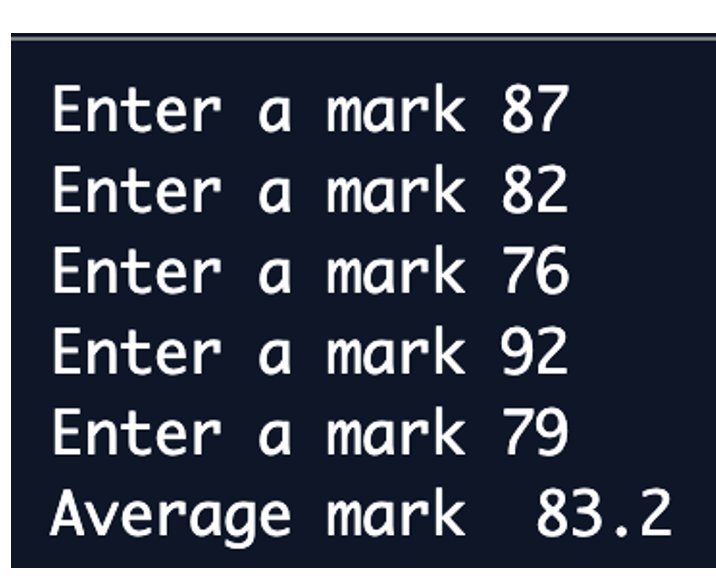# Repetition: For Loop

## The for loop

The for loop repeats a block of code for number of times. In this example, we will repeat.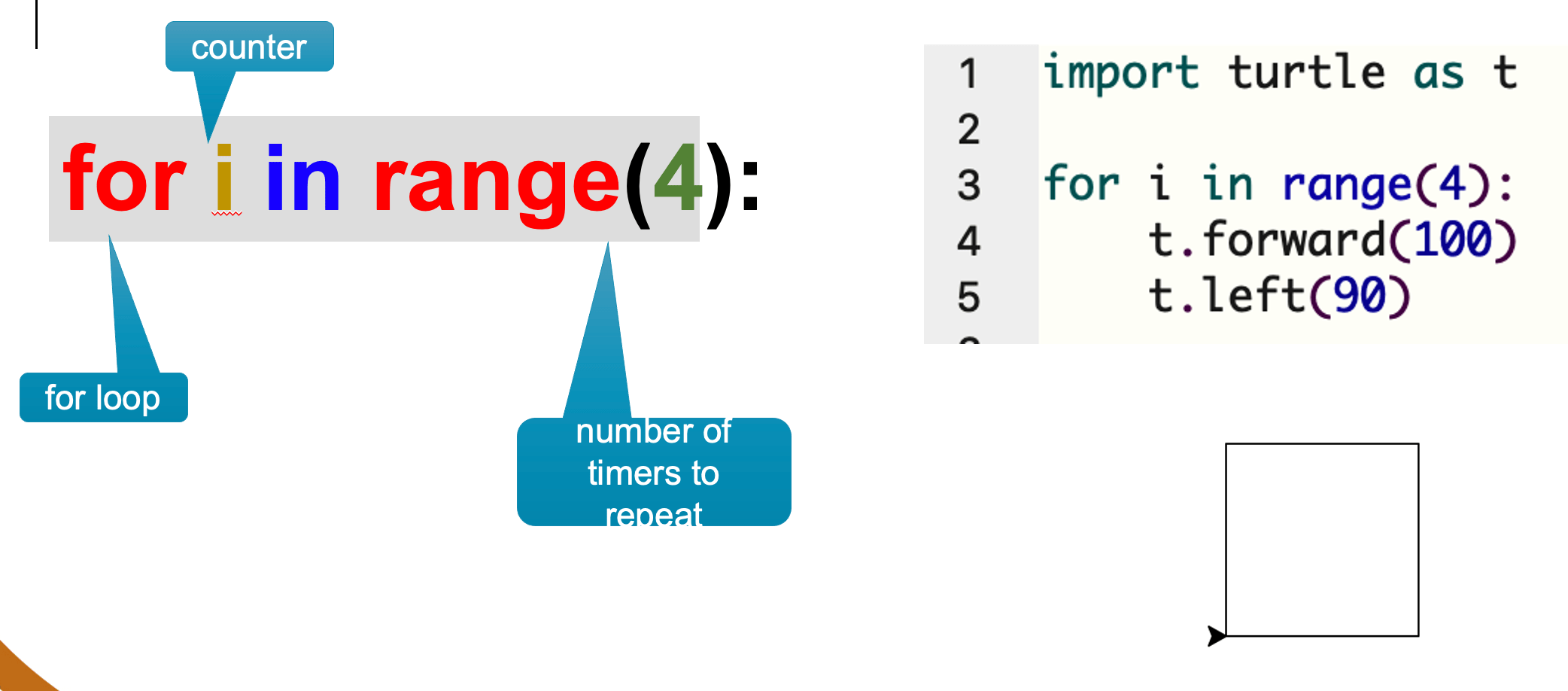## Key points to remember

• The for loop is one kind of loop. It uses a counter which takes on a value every time the loop iterates.
• The range() function indicates how many times the statement will repeat. For example range(4) means repeat 4 times and range(10) means repeat 10 times

## Version 1: For loop with only end value

```	    for i in range(endValue):
Statements
```

The starting value of loop is 0. In actual fact, range(4) generates four numbers 0,1,2 and 3 and the counter takes on each value one at a time.

### Example 1

```	   for i in range (6):
print(i)
```
the output is: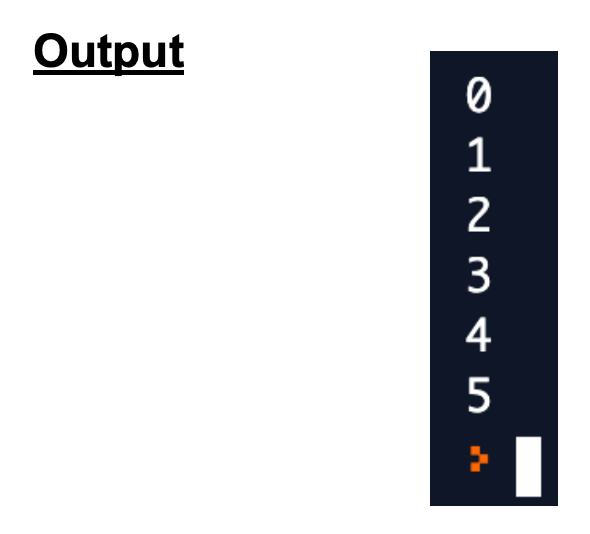## Version 2: For loop with start and end value

```	    for i in range(startValue, endValue):
Statements
```

The starting value of loop can be changed to any given number. Note: Start value must be less than the end value, otherwise loop will not be executed.

### Example 2

```	   for i in range (4:9):
print(i)
print("Outside the loop")
```
the output is: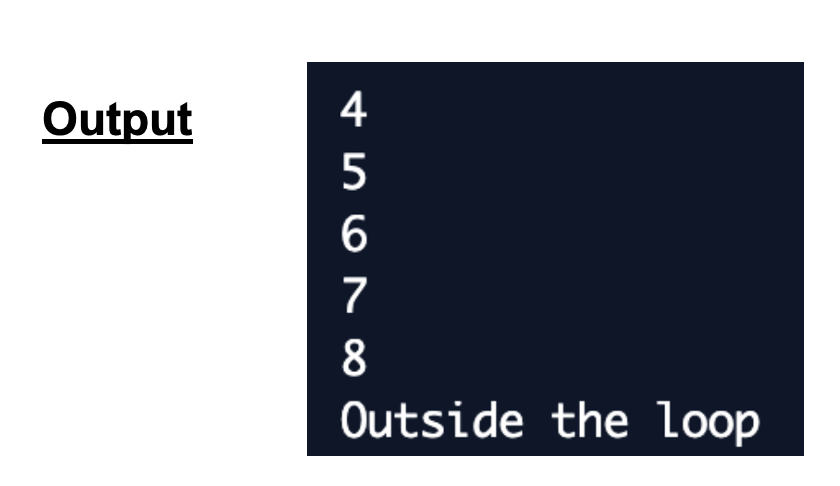h2>Version 3: For loop with increment value
```	    for i in range(startValue, endValue, stepValue):
Statements

```

The starting value of loop can be changed to any given number. Step value can be changed from 1 to any value. Note: Step value must be negative if start value is greater than end value.

### Example 3

```	   print("Display even numbers from 2 to 7")
for i in range (2,7,2):
print(i)
print("Display numbers from 5 to 1")
for i in range (5,0,-1):
print(i)
```
The output is: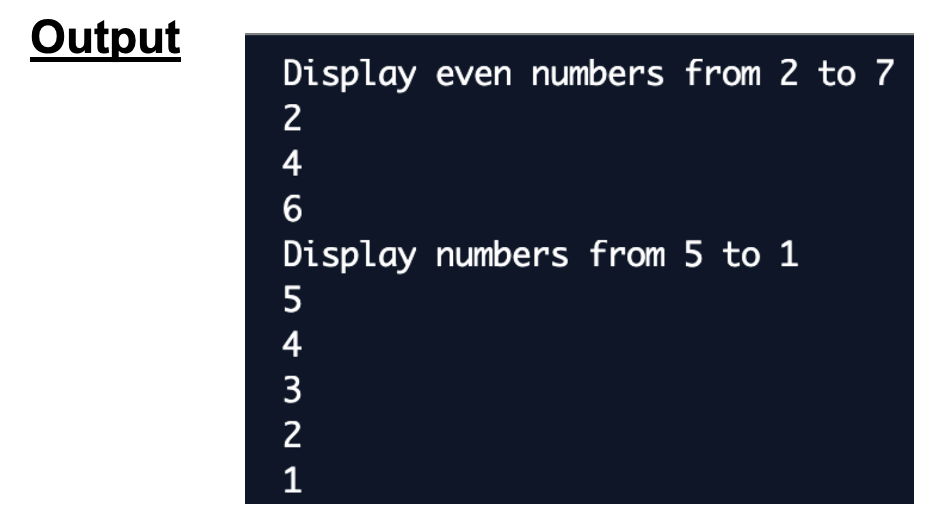### Example using loop

Exercise 1: Write a program that reads 5 marks of a student, calculates and displays the average price.

```	    # program to read 5 marks and display its average
total_marks = 0

for i in range(5):
mark = int(input("Enter a mark "))
total_marks = total_marks + mark

avg_mark = total_marks / 5

print("Average mark ", avg_mark)
```
Note that # indicates a comment line the output is: